Chapter 24

In our book, we make an assumption that all the particles, electrons, protons and neutrons have electromagnetic origin.

We know all the material was made by the molecules, the molecule was consisted of atoms, the atom consists of electrons, protons and neutrons, and so all material has electromagnetic origin.

Electric charge and magnetic charge are the most basic properties of electromagnetic field.

1. Based on the charge distribution, we can get field distribution.
2. Based on the field distribution, we can get field velocity.
3. The charge moves according to the field velocity.
4. Because of the velocity, the charge distribution keeps changing.
5. Because the charge distribution changes, the field then changes.
6. Because the field changes, then the field velocity changes.

Based on the distribution of electric charge and magnetic charge in space, and according the Gauss Law for electric field and magnetic field, we can get the electric field and magnetic field distribution.

And then, based on the electric field and magnetic field space distributions, we can get the electromagnetic field velocity, and then all of the electric and magnetic charges will move according to the electromagnetic field velocity.

The electric charge and magnetic charge space distribution will keep changing, then the electric field and magnetic field will keep changing, and then electromagnetic field velocity will also keep changing.

However the change happened, the electric charge and magnetic charge will keep conserved.

All the basic particles include electrons, protons and neutrons, they are no longer regarded as point-like particles, but are instead considered to have a spherical electromagnetic field with the continuum distribution of electric charge and magnetic charge.

The key relationship between time-space and energy-momentum is the velocity of the electromagnetic field.

As we know, the electromagnetic field velocity has the following relationship with energy-momentum: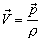(24.1)

In which ‘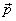’ is the electromagnetic field momentum density, and ‘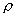’ is the electromagnetic field mass density.

As we know, the electromagnetic field velocity has the following relationship with time-space: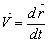(24.2)

We have the following symmetrical relationship between time-space and energy-momentum:

 Field Velocity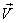Time T Energy E Space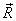Momentum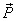Absolute time S Rest energy U

The key between these relations is electromagnetic field velocity.

As we know, the rest energy density ‘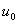’ is defined as: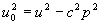(24.3)

In which the electromagnetic field energy density ‘u’ is: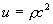(24.4)

In which ‘is the electromagnetic field mass density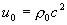(24.5)

In which ‘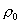is the electromagnetic field rest mass density, thus: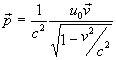(24.6)

Thus: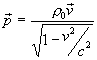(24.7)

The symmetrical part of the rest energy is the absolute time ‘s,’ which is defined as: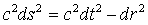(24.8)

Thus the time ‘t’ and the absolute time ‘s’ have following relationship: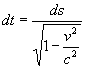(24.9)

The energy always has positive value, and the time is always one-directional.
Time flows from the past into the future along with the direction of field velocity, the arrow of time is the direction of the field velocity.